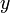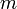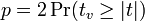# Independent Samples t-Test - Comparing Two Means with Unequal Variances

Jump to: navigation, search

The test statistic is:$t = \frac{\bar{x} - \bar{y}}{\sqrt{\frac{s^2_x}{m} + \frac{s^2_y}{m}}}$

where:$\bar{x}$ and$\bar{x}$ are the average values of variables$x$ and$y$ respectively, where each of these variables represents the data from two independent groups,
the groups have sample sizes of$m$ and$n$ respectively,$s^2_x$ and$s^2_y$ are the variances in the two groups,$p = 2\Pr(t_v \ge |t|)$,$v = \frac{(\frac{s^2_x}{n} +\frac{s^2_y}{m} )^2}{\frac{(\frac{s^2_x}{n / d_{eff}})^2}{n-b}+\frac{(\frac{s^2_y}{m / d_{eff}})^2}{m-b} }$,$b$ is 1 if Bessel's correction is selected for Means in Statistical Assumptions and 0 otherwise,$d_{eff}$ is Extra Deff.